Nishant Malhotra Founder of Middle Road OPC Pvt Ltd & The middle Road platform talks about the startup

### Ahoy Ahoy-Ahoy!

Welcome to the Kickass platform enabling social change & impact

# Understanding Type II Error

Read on the methodology of calculating Type II error for a hypothesis test about a population mean articulated with sufficient examples. The note includes an excel worksheet attached which can be readily downloaded. Understand power curve, the importance of the level of significance on Type I error followed up with a couple of examples on calculating Type II error along with a tutorial explaining concepts  related on this subject matter.

This tutorial builds on the previous tutorials and discusses Type I and Type II errors in hypothesis testing in detail. Type I error (α) occurs when you reject a null hypothesis when it is true. Type I error depends on the level of significance of a hypothesis test also known as alpha (α). As the level of significance increases, the test statistic i.e. the Z value increases and p-value increases. “A 5% alpha means there is that there is a 5% chance of concluding a difference exists when there is no difference and as we proceed we understand this statement.”The Internal Revenue Service (IRS) provides a toll-free help line for taxpayers to call in and get answers to questions as they prepare their tax returns. In recent years, the IRS has been inundated with tax payer calls and has redesigned its phone service as well as posted answers to frequently asked questions on its website (The Cincinnati Enquirer, January 7, 2010).  According to a report by a taxpayer advocate, callers using the new system can expect to wait on hold for an unreasonably long time of 12 minutes before being able to talk to an IRS employee. Suppose you select a sample of 50 callers after the new phone service has been implemented, the sample results show a mean waiting time of 10 minutes before an IRS official comes on a line. Based upon data from past years, you decide it is reasonable to assume that the standard deviation of waiting time in 8 minutes. Using your sample results, can you conclude that the actual mean waiting time turned out to be significantly less than 12-minute claim made by the taxpayer advocate? Statistics for Business & Economics by Anderson | Sweeney | Williams | Camm | Cochran

Use alpha =0.05Here the population parameter given is 12 minutes that is the population mean. Recall the null hypothesis always equates to the status quo, which means your null hypothesis will always be 12 or more. Another hint in the question is the following words “can you conclude that the actual mean waiting time turned out to be significantly less than the 12-minute claim made by the taxpayer advocate”. The alternative hypothesis is the research hypothesis that is mentioned within the exclamation marks. This is the biggest hint- the alternative hypothesis is less than 12 minutes. Whenever you are confused about the null hypothesis it’s good to look at the alternative hypothesis. This is an excellent example to understand the meaning of level of significance and Type I error. This is a single tailed test.

Once you have done with the hypothesis test, you need to understand the next step to set up the test statistic. A sample of 50 callers analysis shows an average of 10 minutes with a standard deviation of 8 minutes. The Z value is the following.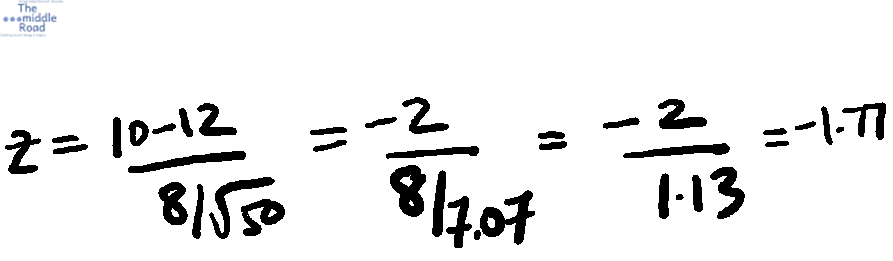The level of significance is 0.05 or 5% which means the probability of making a Type I error is 5% i.e. accepting the alternative hypothesis when the null hypothesis is correct. Using Norm. S. Dist function we get the p-value of 3.84%. Since the p-value is less than 5%, we can accept the alternative hypothesis concluding that the actual meantime turned out to be significantly less than the 12 min claim by the tax advocate. But let’s hold our horses and now go beyond the question. Take alpha as 0.01 or 1%. At a level of significance of 1%, the picture is a bit different. the p-value is greater than 1% i.e. 3.84 so you cannot accept the alternative hypothesis. As the level of significance reduces the probability of making a Type I error reduces as the probability of concluding there is a difference when there is none reduces. Null hypothesis underlining assumption is that there is no difference, now we understand how reducing the level of significance means limiting the Type I error. When it’s utmost critical to control Type I error, reduce the level of significance. Check out the tutorial section here.

Reducing level of significance also increases the likelihood of  Type II error i.e. accepting null hypothesis when it is not true. In many cases, there are two outcome with different decisions. This makes it more important to understand the probability of making a Type II error. Refer to the tutorial for more examples.

### Calculating the probability of Type II error for a hypothesis test about a population mean Understanding Decision making through statisticsThe following section will guide how to calculate Type II error for a hypothesis test about a population mean.

In the below pic, there are two decisions to be met depending on the outcome of the hypothesis test. Usually you will encounter these when you want to accept a service or a product based on a parameter meaning if the parameter is met you accept the service or product otherwise reject it. Taking example of the airline industry. While selecting airplanes you must adhere to the strictest guidelines.

Example if the quality standards must be met at a particular level, we must take the probability of a Type II error into account. The below example would help in understanding the methodology in calculating the probability of Type II error for a given population mean.

Beta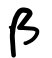is Type II error and 1-beta is the probability of correctly rejecting the null hypothesis. The tutorial section is here.

The below example is from the book Statistics for Business & Economics by Anderson | Sweeney | Williams | Camm | Cochran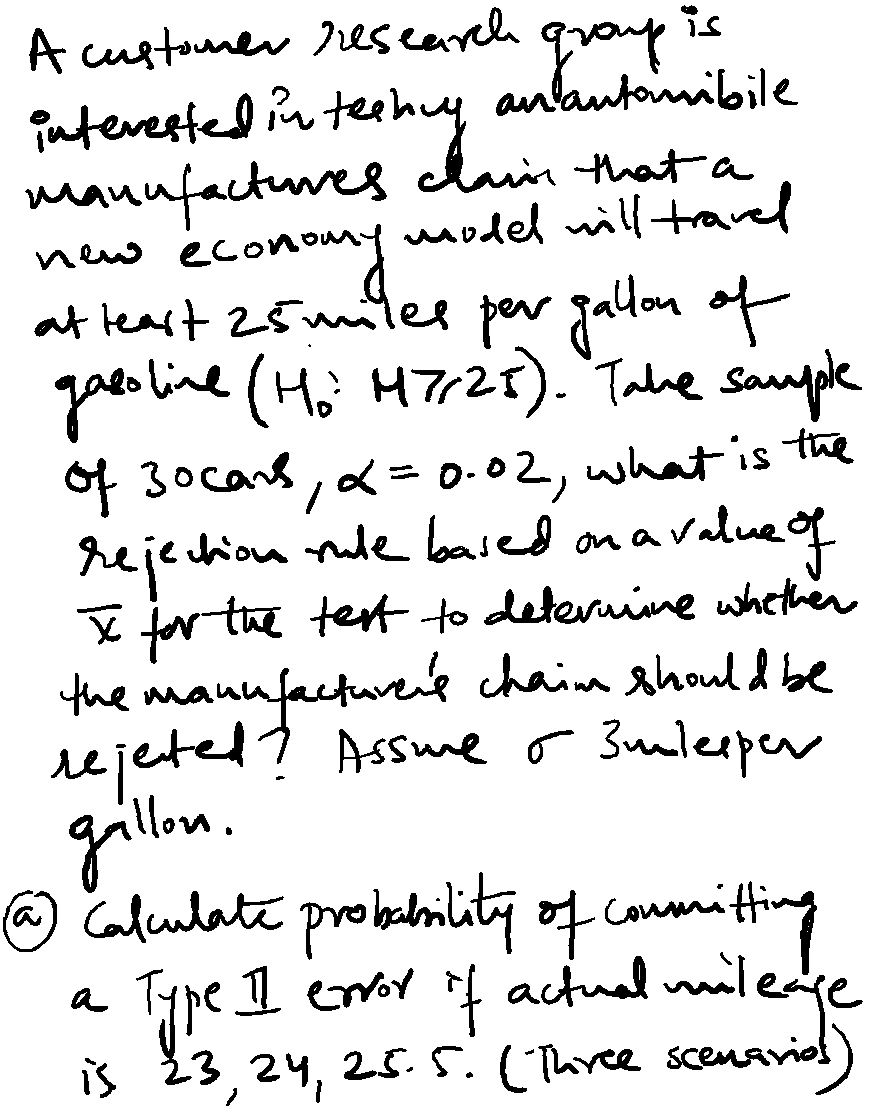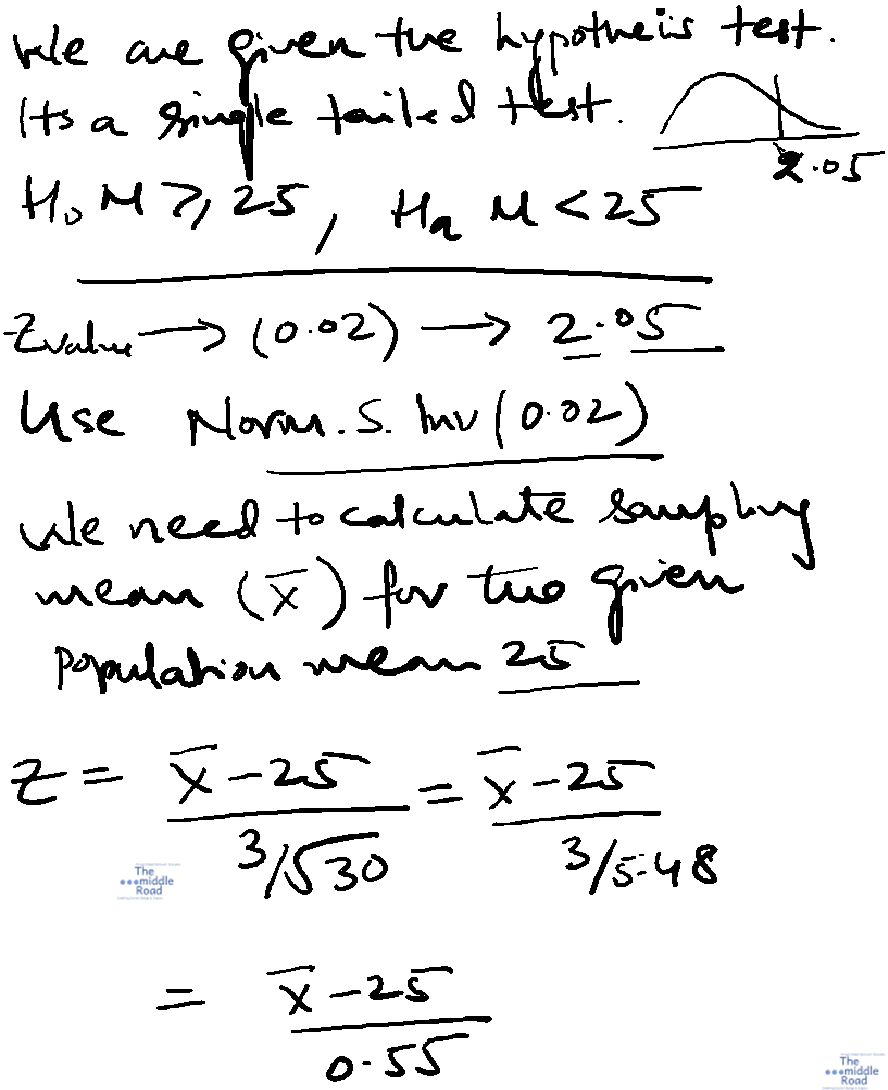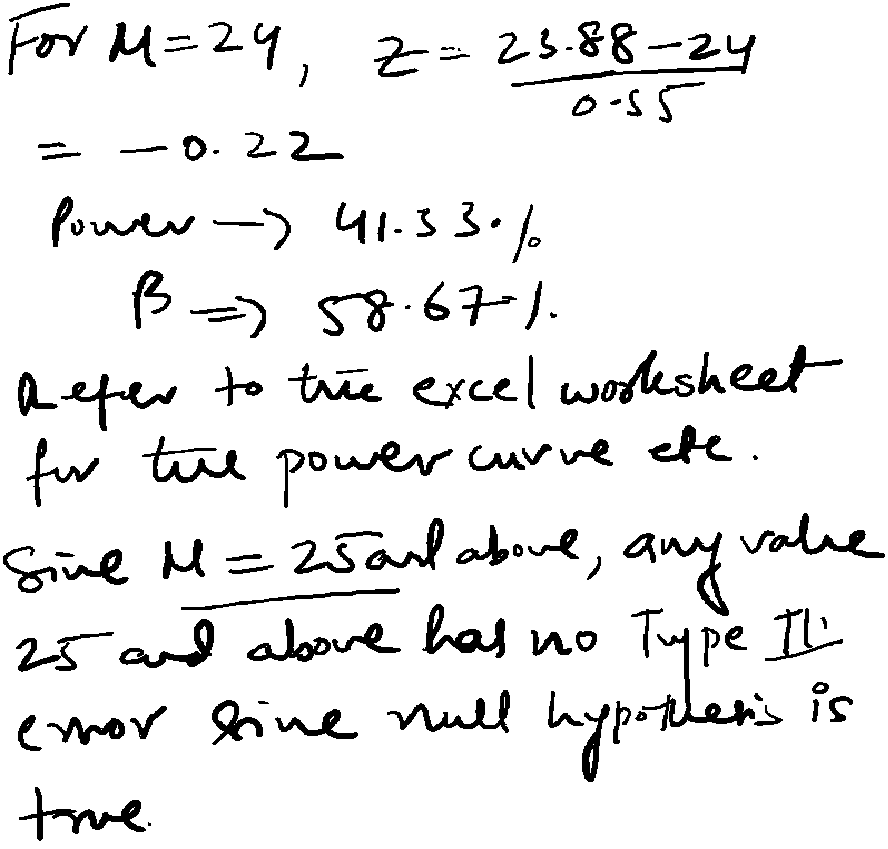Lets look at another example from the book Statistics for Business & Economics by Anderson | Sweeney | Williams | Camm | Cochran. In this example we will use the concept of confidence interval to estimate  probability of Type II error.

a. In this case, to assume the population mean is equal to 28 when it is not.

Lets begin by making a confidence interval for alpha =0.05. This is a two tailed test, so  calculate the Z value for alpha =0.025. Norm.S.Dist(0.025) will give the Z value as +/- 1.96.  The two points of rejection is 0.25 and 0.75.  For a two tailed test the level of significance is 0.05/2=0.025.

The p value for 1.37 is 0.9147. Now substitute 29.176 in the equation, Z= 29.176- 26/0.6 = 3.176 the corresponding p value is 1. The probability of accepting the null hypothesis is p-value (3.176) – p-value (1.37) = 1-0.9147=0.0853 = 8.53% This also the power of population mean value 26.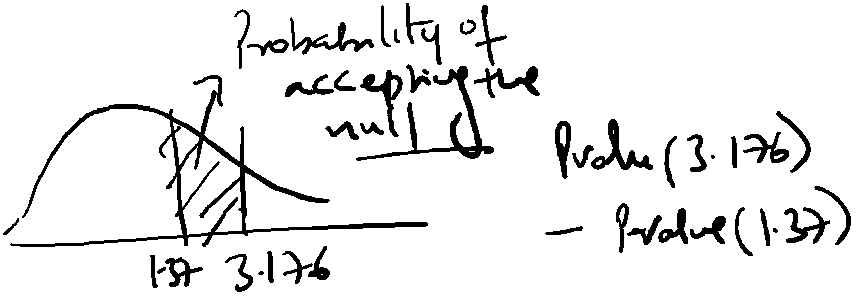For 27, calculate Z= 26.842-27/0.6 = -0.293 p value 0.3846 and Z= 29.176-27/0.6= 0.9998, the probability of accepting the null hypothesis is 0.9998-0.3846 = 0.6152. In the book this is 0.6179. Calculate the rest using the process outlined.